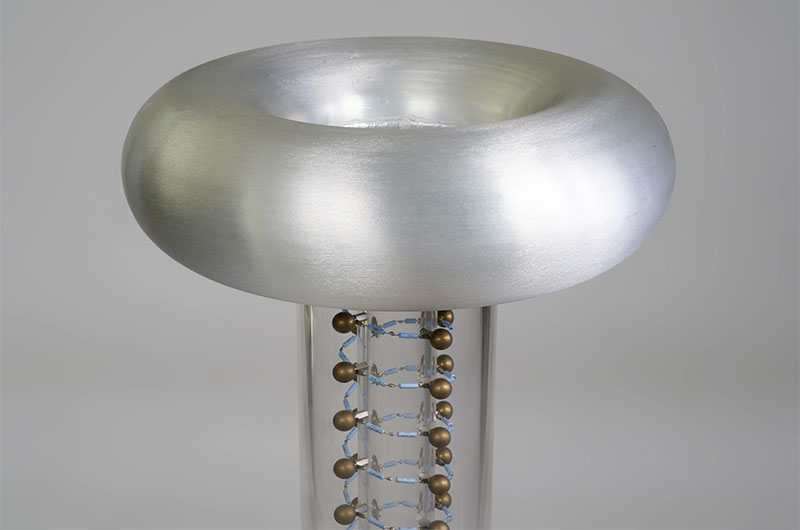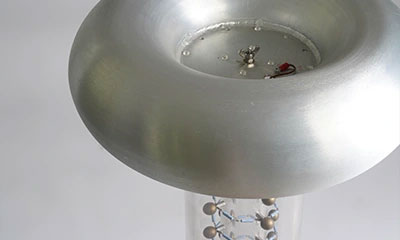# Tech Tips## About the basics of voltage measurement

Voltage measurements are made to ensure that electrical equipment is working properly and to check electrical circuits. When measuring voltage, connect the terminal of the voltmeter in parallel with the part you want to measure.

If the voltage is in the range of 1 V to 10 V, such as "circuit used in school experiments" or "battery-powered toys," you can easily measure it with a voltmeter or tester. However, when the voltage is high or conversely low, you cannot measure it as usual. In that case, special equipment and methods are required.

## How to measure high voltage (1000 V or more)

When measuring a high voltage of 1000 V or higher, you cannot measure it directly with a standard measuring instrument. High voltage has so much energy that it destroys standard measuring instruments. There is also a risk of electric discharge, and you must take electric shock, so great care.

The basic idea of high-voltage measurement is to "connect a device with a high resistance value in series to the outside of the measuring instrument." The voltage across the series of resistances is proportional to the magnitude of each resistance according to Ohm's law. The voltage applied to the voltmeter will be distributed if you connect a voltmeter and a high resistance in series.

For example, if the ratio of the connected resistance to the internal resistance of the measuring instrument is 9 : 1, the voltage applied to the resistance and the measuring instrument will also be 9 : 1. Compared to the state where no resistor is connected, the voltage applied to the measuring instrument is 1 / 10. This method is called voltage divided, and there are the following methods for measuring high voltage using this mechanism.

### The voltage divider (high voltage divider)

The voltage divider has a circuit built into the housing, an input terminal, and a measurement terminal. Also called a high-voltage divider, the basic circuit diagram of the voltage divider is as follows. It is used for measuring DC voltage, but it is also possible to measure AC voltage if the frequency supplied by the electric power company is 50 Hz or 60 Hz.

Note that the voltage divider may generate heat during use, so you must take care to change the resistance value due to heat. It is also necessary to consider the voltage divider's resistance and the voltmeter's input resistance when making measurements. Since it handles high voltage, be careful not to leak from dirt or foreign matter adhering to the terminals.Voltage divider configuration circuit### Multiplier

Since the principle of the multiplier is the same as that of the voltage divider, the precautions for use are also the same. However, the voltage divider can measure AC voltage in some frequency bands, but the multiplier can only measure DC current.

### High voltage probe

Use a probe when measuring voltage waveforms with an oscilloscope. There are various types of probes for voltage measurement, including those that enable accurate measurement of high-frequency signals and those that reduce the effect on the measurement target. Among them, the probe that supports high voltage is the high voltage probe.

High voltage probes have different measurable voltages depending on the frequency. Therefore, be sure to check not only the maximum input voltage but also the frequency and the voltage that can be applied.

When measuring a high AC voltage, there is also a method of changing the voltage using the induced electromotive force of the coil.

### VT (Voltage Transformer)

VT (Voltage Transformer) is a device that converts the high voltage of an AC circuit into a voltage that is easy to measure. Previously it was called PT (Potential Transformer). The basic structure of the VT is the same as that of a transformer, as shown in the figure below. The coil on the input side and the coil on the output side change the number of turns and are wound around a donut-shaped iron core. The overhead wire voltmeter for railways is also a member of this group. Please note that the error tends to be large depending on the voltmeter to be combined.

### Static electricity measuring instrument (Electrostatic fieldmeter)

Static electricity is a typical example of electricity with high voltage. Since static electricity cannot be accurately measured when it comes into contact with a probe, etc., you must use a non-contact measuring instrument. A voltmeter for measuring static electricity is called an electrostatic voltmeter, and there are types such as surface electrometers and electrostatic fieldmeter.

It is a device that measures static electricity on the surface by moving the pointer by the force that the two electrodes are attracted to or repelled by the static-charged object to be measured. The feature is that it can be measured by itself without other measuring instruments.

High-voltage circuits can cause a discharge even if they are not in contact with each other or lead to a serious accident if there is an electric leakage. Safety measures such as proper grounding are also necessary when measuring high voltage. Follow the correct usage according to the specifications of the device.

## How to measure low voltage (1 mV or less)

You cannot measure even a voltage of 1 mV or less with a regular measuring instrument. Contrary to the high voltage, it is necessary to perform electrical amplification for measurement.

Also, at low voltages, the original voltage is low, and the waves are small, so the noise that would not usually be a problem will significantly affect the measurement results. Noise removal is an important issue when measuring low voltages.

Noise that affects voltage includes external noise such as electromagnetic waves generated when the surrounding electrical equipment is switched on and off, as well as internal noise generated in the measurement circuit.

The points to remove noise in both direct current (DC) and alternating current (AC) are as follows.

### In the case of DC

In circuits containing different metals, different temperatures at the junctions generate electromotive forces. This phenomenon is called Thermal Electromotive Force (EMF). Even in circuits that measure voltage, multiple types of metals are used in terminals, connectors, solder, and so on. Therefore, if there is a difference in the temperature of these joints, it will generate the thermal electromotive force.

To make it difficult to generate the thermal electromotive force, do not use conductors made of different materials; if you touch the terminal, wait for the terminal to cool down before measuring, air conditioning, or adjusting the ambient temperature.

### In the case of AC

One thing to keep in mind when measuring low voltage alternating current is electromagnetic induction. In alternating current, the direction of the current is constantly changing, so electromagnetic induction occurs around the lead wire. It is a good idea to reduce the input resistance of the measuring instrument or use one with two lead wires twisted.

In low voltage measurements, even small factors that are not usually a problem can become noise and affect the measurement. For example, the effect of static electricity cannot be ignored. If there is an electric charge such as the measurer's clothing nearby, it may cause noise.

It is also necessary to shield the measurement environment and prepare an environment where static electricity is unlikely to occur. Pay attention not only to the measuring equipment and circuits but also to the surrounding environment.